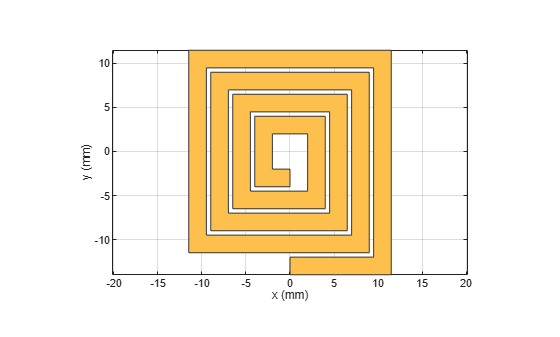# traceSpiral

Create even-sided polygon trace in spiral form

## Description

Use the `traceSpiral` object to create an even-sided polygon trace such as a square, hexagon, octagon, decagon, or a circle in a spiral form.

## Creation

### Syntax

``trace = traceSpiral``
``trace = traceSpiral(Name=Value)``

### Description

example

````trace = traceSpiral` creates a square spiral trace. The spiral trace is centered at the origin on the X-Y plane.```
``` `trace = traceSpiral(Name=Value)` sets Properties using one or more name-value arguments. For example, `traceSpiral(ReferencePoint=[1 1])` creates a spiral trace with the reference point `[1 1]`. Properties not specified retain their default values.```

## Properties

expand all

Name of the spiral trace, specified as a character vector or a string scalar.

Example: `trace = traceSpiral(Name="spiraltrace1")`

Data Types: `char` | `string`

Point of reference of the spiral trace in Cartesian coordinates, specified as a two-element vector. Use the reference point to modify the shape from its initial position.

Example: `trace = traceSpiral(ReferencePoint=[1 1])`

Data Types: `double`

Inner diameter of the spiral trace in meters, specified as a positive scalar. If the polygon is a square, the inner diameter is the distance between the innermost vertex and the midpoint of the opposite side of the inner square. For all other shapes, the value is the distance between the innermost vertex and the opposite vertex of the inner turn.

Example: `trace = traceSpiral(InnerDiameter=0.0015)`

Data Types: `double`

Width of the spiral trace in meters, specified as a positive scalar.

Example: `trace = traceSpiral(TraceWidth=0.0050)`

Data Types: `double`

Distance between the traces of the spiral in meters, specified as a positive scalar. For a square spiral trace, the spacing is the gap between the flat edges of adjacent turns. For all other shapes, the spacing is the gap between vertices of adjacent turns.

Example: `trace = traceSpiral(Spacing=6.0000e-04)`

Data Types: `double`

Number of turns in the spiral, specified as a positive scalar.

Example: `trace = traceSpiral(NumTurns=6)`

Data Types: `double`

Number of sides in each turn of the spiral based on the polygon, specified as a positive, even scalar. The minimum number of sides is `4` and the maximum number is `10`. If the number exceeds `10`, then the shape is a circle.

Example: `trace = traceSpiral(NumSides=6)`

Data Types: `double`

## Object Functions

 `add` Boolean unite operation on two RF PCB shapes `and` Shape1 & Shape2 for RF PCB shapes `area` Calculate area of RF PCB shape in square meters `intersect` Boolean intersection operation on two RF PCB shapes `mesh` Change and view mesh properties of metal or dielectric in PCB component `minus` Shape1 - Shape2 for RF PCB shapes `plus` Shape1 + Shape2 for RF PCB shapes `rotate` Rotate RF PCB shape about defined axis `rotateX` Rotate RF PCB shape about x-axis `rotateY` Rotate RF PCB shape about y-axis and angle `rotateZ` Rotate RF PCB shape about z-axis `subtract` Boolean subtraction operation on two RF PCB shapes `scale` Change size of RF PCB shape by fixed amount `show` Display PCB component structure or PCB shape `translate` Move RF PCB shape to new location

## Examples

collapse all

Create a spiral trace with default properties.

`trace = traceSpiral`
```trace = traceSpiral with properties: Name: 'myspiral' ReferencePoint: [0 0] InnerDiameter: 0.0040 TraceWidth: 0.0020 Spacing: 5.0000e-04 NumTurns: 4 NumSides: 4 ```

View the trace.

`show(trace)`## Version History

Introduced in R2021b